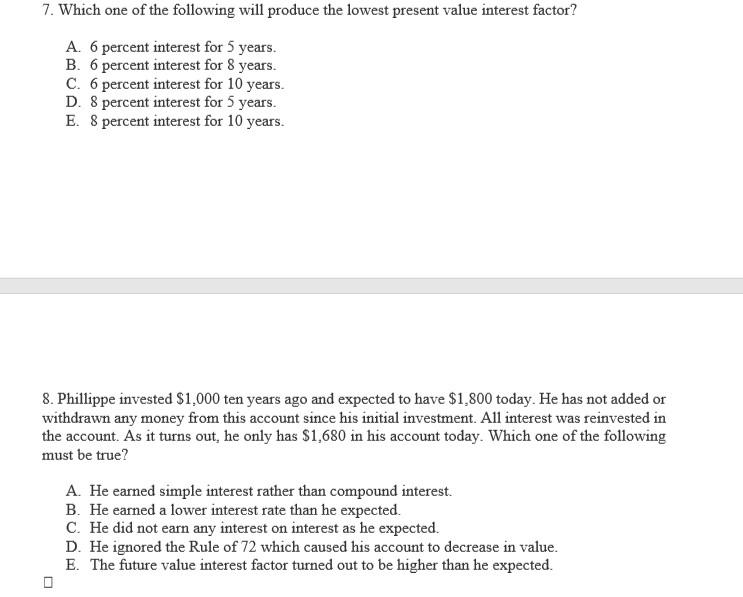# Which one of the following will produce the lowest present value interest factor?7. Which one of the following will produce the lowest present value interest factor? A. 6 percent interest for 5 years. B. 6 percent interest for 8 years. C. 6 percent interest for 10 years. D. 8 percent interest for 5 years. E. 8 percent interest for 10 years. 8. Phillippe invested \$1,000 ten years ago and expected to have \$1,800 today. He has not added or withdrawn any money from this account since his initial investment. All interest was reinvested in the account. As it turns out, he only has \$1,680 in his account today. Which one of the following must be true? A. He earned simple interest rather than compound interest. B. He earned a lower interest rate than he expected. C. He did not earn any interest on interest as he expected. D. He ignored the Rule of 72 which caused his account to decrease in value. E. The future value interest factor turned out to be higher than he expected.

1.
Present value interest factor decreases with rise in rates and
increase in time
Option E
2.
Option B